## LSTM原理及Pytorch搭建LSTM网络实验

LSTM是一种特殊的RNN，其引入状态量来保留历史信息，同时引入门的概念来更新状态量。

RNN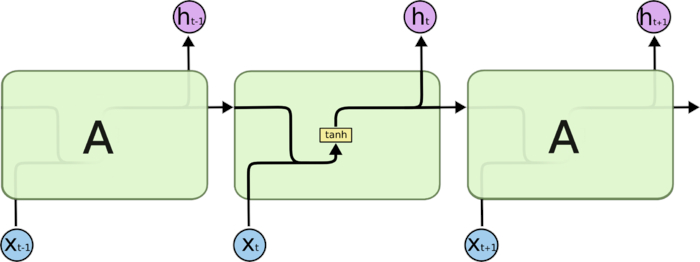LSTM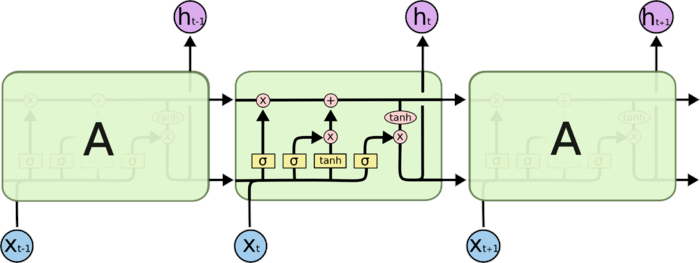RNN网络中历史信息在每个RNN单元，都经过tanh/ReLu，信息在逐渐流失；而LSTM，采用信息更新的方式，更容易将有用的信息传递下去，传得更远。也就是下图中C随序列传递的过程。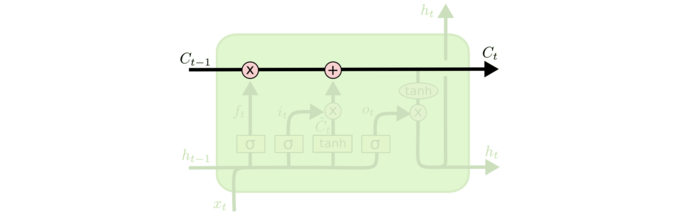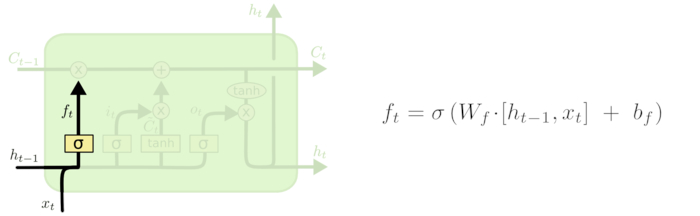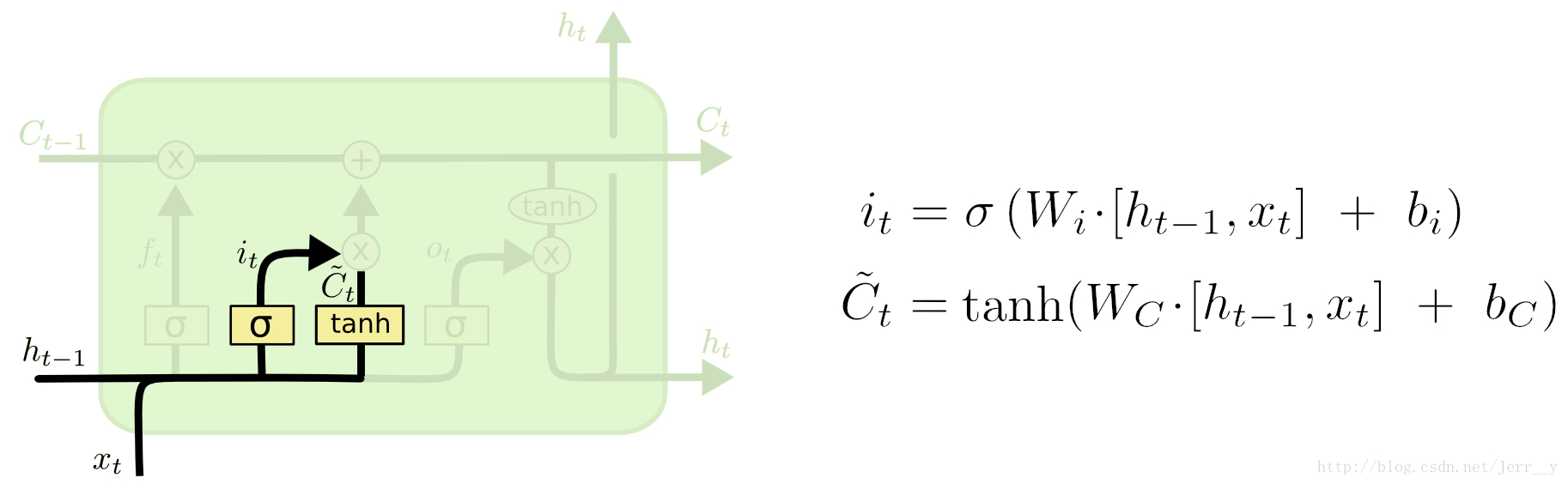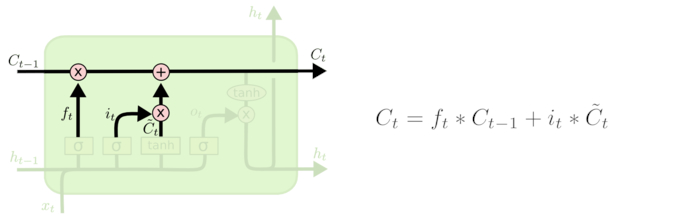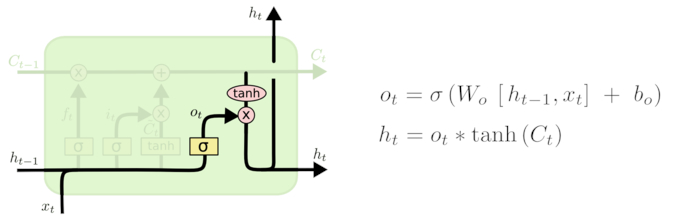LSTM变体GRU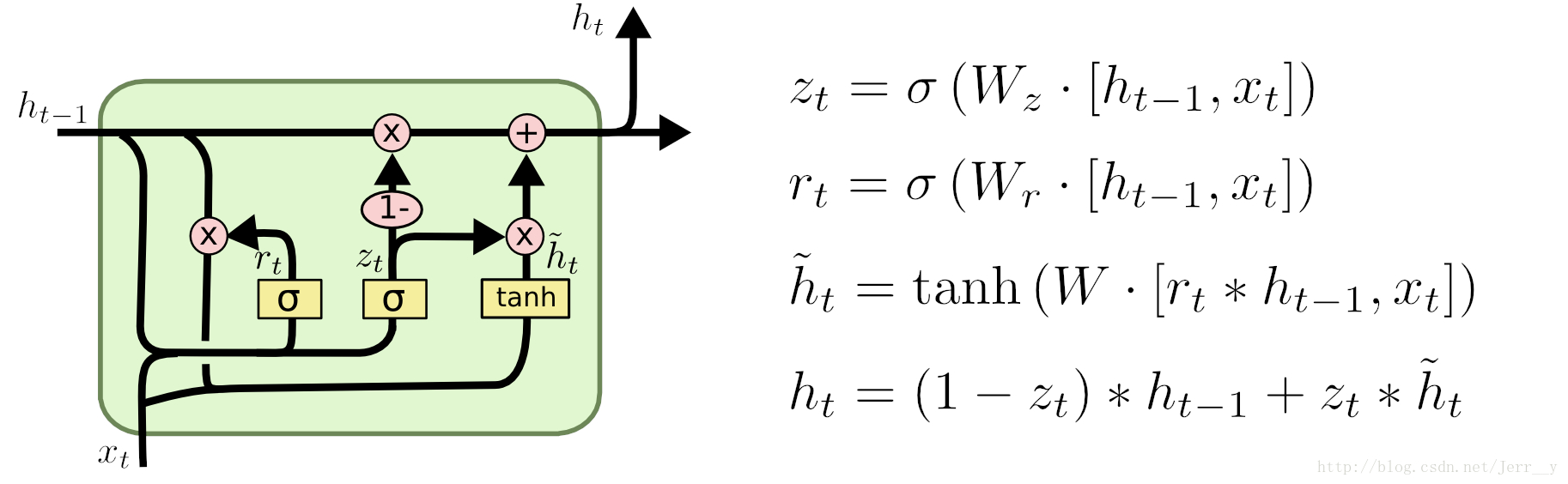1) GRU 少一个门，同时少了细胞状态Ct。

2) 在 LSTM 中，通过遗忘门和传入门控制信息的保留和传入；GRU 则通过重置门来控制是否要保留原来隐藏状态的信息，但是不再限制当前信息的传入。

3) 在 LSTM 中，虽然得到了新的细胞状态 Ct，但是还不能直接输出，而是需要经过一个过滤的处理；同样，在 GRU 中, 虽然我们也得到了新的隐藏状态h^t， 但是还不能直接输出，而是通过更新门来控制最后的输出：ht=(1−zt)∗ht−1+zt∗h^t

LSTM变体FC-LSTM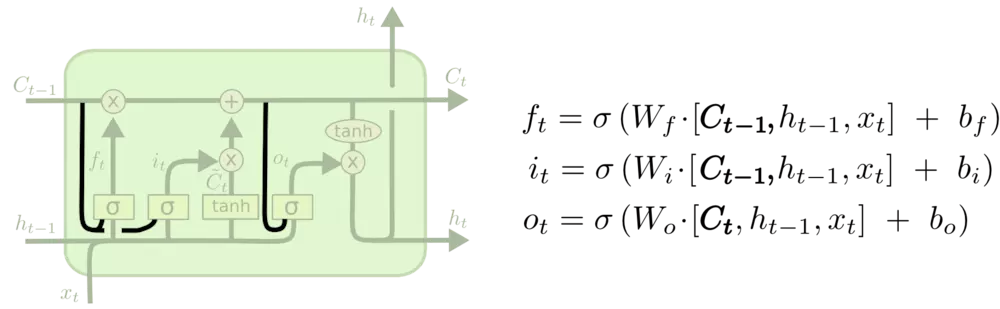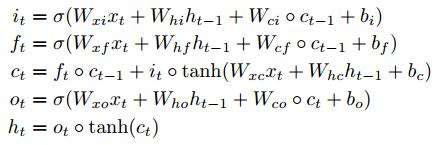import torch
from torch import nn
import numpy as np

class Rnn(nn.Module):
def __init__(self, INPUT_SIZE):
super(Rnn, self).__init__()

self.rnn = nn.LSTM(
input_size=INPUT_SIZE,
hidden_size=32,
num_layers=2,
batch_first=True
)

self.out = nn.Linear(32, 1)

def forward(self, x, hc_state):
# input(x): batch, seq_len, input_size = 1, 10, 2
# output(r_out): batch, seq_len, hidden_size * num_directions = 1, 10, 32*1
r_out, hc_state = self.rnn(x, hc_state)

outs = []
for time in range(r_out.size(1)):
outs.append(self.out(r_out[:, time, :]))
return torch.stack(outs, dim=1), hc_state

# 定义一些超参
TIME_STEP = 10
INPUT_SIZE = 2
LR = 0.02
# “看”数据
# plt.plot(steps, y_np, 'r-', label='target(cos)')
# plt.plot(steps, x_np, 'b-', label='input(sin)')
# plt.legend(loc='best')
# plt.show()

# 选择模型
model = Rnn(INPUT_SIZE)
print(model)

# 定义优化器和损失函数
loss_func = nn.MSELoss()

h_state = torch.autograd.Variable(torch.zeros(2,1,32)) # h0/c0: num_layers * num_directions, batch, hidden_size = 2*1, 1, 32
for step in range(300):
start, end = step * np.pi, (step+1)*np.pi

steps = np.linspace(start, end, TIME_STEP, dtype=np.float32)
x_np = np.sin(steps)
y_np = np.cos(steps)

x = torch.from_numpy(x_np[np.newaxis, :, np.newaxis])
y = torch.from_numpy(y_np[np.newaxis, :, np.newaxis])

# 为了演示，重复x将输入数据特征扩展为两维
prediction, (h_state, c_state) = model(torch.cat((x,x), 2), (h_state, c_state))
h_state = h_state.data
c_state = c_state.data

loss = loss_func(prediction, y)
loss.backward()
optimizer.step()
print("x:")
print(x)
print("y:")
print(y)
print("predict:")
print(prediction)

◎欢迎参与讨论，请在这里发表您的看法、交流您的观点。

[查看权限]